## Note

This is my undergraduate assignment that I translated to English myself in the Electrical Measurement course where the task is to write an essay on AVO Meters. This assignment has never been published anywhere and I, as the author and copyright holder, license this assignment customized CC-BY-SA where anyone can share, copy, republish, and sell on condition to state my name as the author and notify that the original and open version available here.

## Chapter 1 Introduction

### 1.1 Background

In this day and age (2011), the name AVO meter is familiar. Almost all households have an AVO meter.

Now every household cannot be separated from the use of electricity. In this modern era, almost all activities require electric power. Pumping water with an electric pump, cooling food and preserving it using the refrigerator, watching television, moreover at night you have to turn on the lights, all of that needs electricity. If there are problems with power tools, first look at the damage visually. If it doesn't work, then you need an electricity measurement using an AVO meter.

Not necessarily everyone can use the AVO meter, maybe in a household, mostly only 1 person can use it and know what it is. Few also know how it works (only those who specifically learn about electricity).

### 1.2 Objective

The purpose of writing this paper is to explain to ordinary people about AVO meters and how to use them.

### 1.3 Scope of Material

1. Definition of AVO meter.
2. History of the AVO meter.
3. How the AVO meter works.
4. How to use the AVO meter.
5. AVO meter development.

## Chapter 2 Basic Theory

### 2.1 AVO Meter

AVO stands for Ampere Volt Ohm. The meter here can be defined as a measuring instrument. AVO meter is a tool that can measure current (amperes), voltage (volts), resistance (ohms).

### 2.2 Charge (Coulumb)

In this world, there are 2 charges, namely positive and negative charges. There is also a neutral charge, where the molecule has the same positive and negative power. If the load is like (++, --) will reject reject. When the charges are different (+ -) will be of attraction. The force that occurs between charges is called the coulomb force.

Electricity can be generated by flowing charges. Take the salt bridge, for example. The electrons flowing into the protons are called electric currents.

### 2.3 Current (Ampere)

Current is defined as charge flowing in units of time.

I = dq/dt, dq = q2 - q1, dt = t2 - t1

I = Current (ampere)

q = Charge (coulomb)

t = Time (second)

To make it easy to understand the formula above, it can be said that the amount of coulomb that flows every second. The person who contributed to the discovery of currents was a mathematician and physicist from France, namely André-Marie Ampère.

### 2.4 Voltage (Volt)

The voltage in electricity can be said to be the amount of energy carried by each charge.

V = dw/dq

V = voltage (volt)

W = enegy (joule)

q = charge (coulomb)

For easy understanding this formula can be said of the energy contained per 1 coulumb. The name of the volt comes from the Italian physicist Count Alessandro Giuseppe Antonio Anastasio Volta.

### 2.5 Resistansi (Ohm)

German physicist George Simon Ohm found that the voltage applied to an object is directly proportional to the current flowing. Each object has a different resistance.

For example, for the first object, if given 2V it will flow 1A, if given 4V it will flow 2A. The first object has a resistance of 2Ω. For the second object if given 4V will flow 5A, if given 8V will flow 10A. The second object has a resistance of 4/5Ω.

V=IR, I=V/R, R=V/I

V = voltage (volt)

I = current (ampere)

R = resistance (ohm)

Resistance is an electrical resistance which can be said to be the amount of voltage required to flow a current.

### 2.6 AC and DC voltage

DC stands for direct current which means a straight flowing current. AC stands for alternating current, which means the current that flows up and down following a sine wave.

## Chapter 3 Discussion

### 3.1 Question

1. Before discussing the AVO meter, you should know about the Ammeter (Ammeter), Voltmeter and Ohmmeter. What is the definition of Ammeter, Voltmeter and Ohmmeter?
2. What are the basic materials for making an AVO meter or Ammeter, Voltmeter and Ohmmeter?
3. How is it organized?
4. How does it work?
5. How to use it?

### 3.2 Answer

1. Ammeter is a tool for measuring electric current. The unit of electric current is in amperes. Voltmeter is a tool for measuring electric voltage or it can be called the electric potential difference. The unit of electric voltage is the unit of volts. Ohmeter is a tool for measuring resistance to electricity. The unit of resistance is ohms.
2. The basic material for making Ammeter, Voltmeter and Ohmmeter is a Galvanometer. Galvanometer is a tool to detect the presence of electricity. Materials needed to make a simple Galvanometer:
1. North Pole permanent magnet.
2. South Pole permanent magnet.
3. Iron core.
4. Spiral springs.
5. Wire Coils.
6. Wire.
7. Pointing Needle.
Galvanometer itself is a type of ammeter, to make a simple ammeter the above materials are needed. To make the voltmeter, only needed to connect the resistors that are agreed upon internationally. For the ohmmeter, only a voltage generator (battery) is installed to be emitted to the instrument to be measured.

3. The arrangement of the galvanometer is as follows: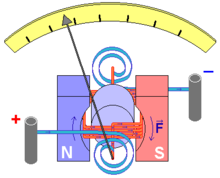D'Arsonval/Weston galvanometer movement.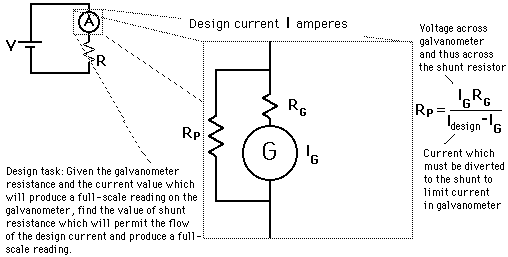The ammeter arrangement is different from the explanation above.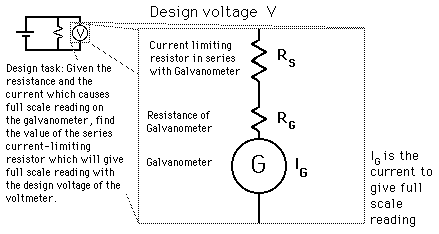Voltmeter arrangement.Ohmmeter arrangement.

4. Galvanometer works by entering electricity into it. After the electricity flows, it will produce a magnetic field that attracts the wire and attracts the spiral spring as well. So that the needle will rotate, and the spring will return it to normal condition after usage.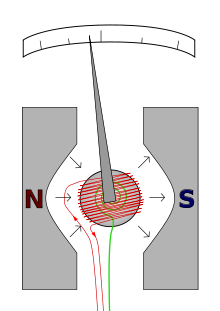D'Arsonval type moving coil galvanometer.
• The red wire carries the current to be measured.
• The recovery spring is shown in green.
• N and S are the north and south poles of the magnet.

Ammeter works on the principle of water flow.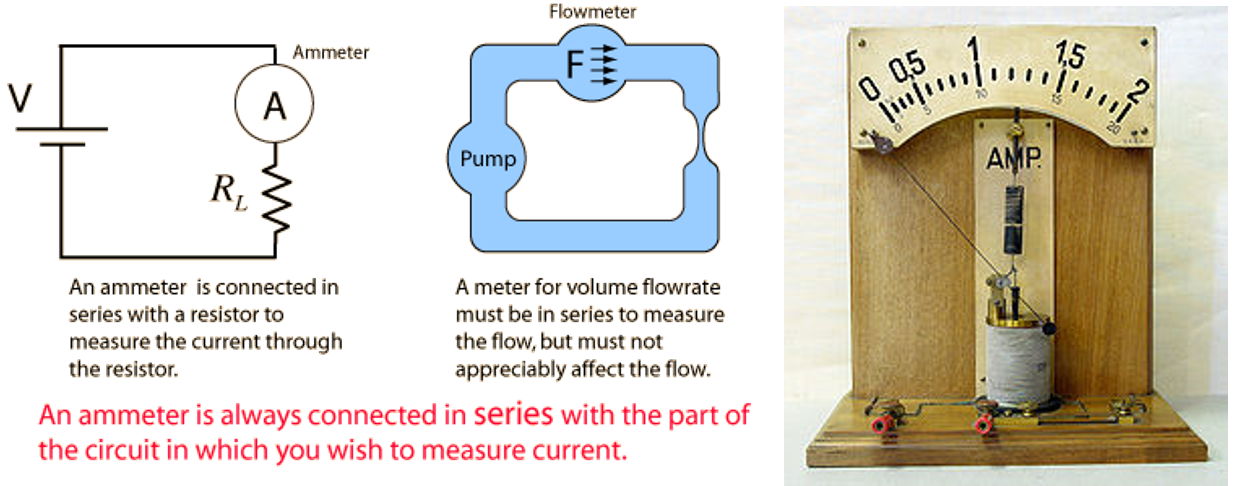Demonstration model of a moving iron ammeter. As the current through the coil increases, the plunger is pulled further into the coil and the pointer turns right.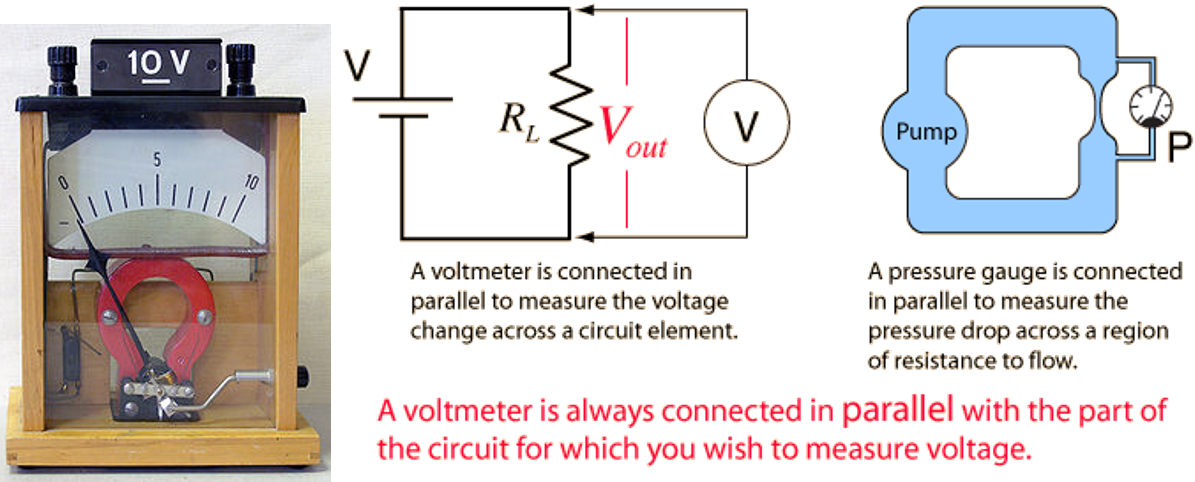For the voltmeter, two poles are added to enter electricity. The goal is to measure the potential bed. Think of it like measuring water pressure.

For the ohmmeter the voltage will be given to the object to be measured its resistance. By applying stress, the resistance will be seen.

5. This section is taken from Putu Rusdi Ariawan's paper on AVO meters, Faculty of Engineering, Udayana University.

#### How to measure DC Voltage

1. Place the selector switch in the DC voltage position (V=)
2. Choose measurement limits (1.5, 5, 10, 50, 150, 500). Where a limit equal to or greater than the voltage to be measured must be selected. For example, the voltage to be measured is 6.5V, then the measuring limit that must be chosen is 10V. Should not choose a smaller limit, because the needle will move past the maximum limit and can damage the moving coil.
3. Connect the probe cable to the power source, the red wire to the positive and the black wire to the negative. This method of installation is called parallel connection. If the wiring polarity is reversed, the meter will move left
4. Read the scale board according to where the needle stops. The most appropriate way to read is perpendicularly where the needle must appear in line with the image of the needle on the reflecting mirror, so there is no reading error (parallax)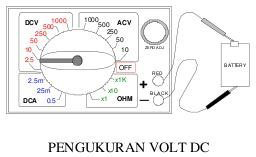DC Volt Measurement

#### Measure AC Voltage

1. Put the selector switch (selector switch) in the position of AC voltage (V˜)
2. Choose a measurement limit (1, 3, 10, 30, 100 or 300). The selected measuring limit must be the same or greater than the voltage to be measured, for example the voltage to be measured is 220V, then the measuring limit that must be selected is 300 V. You cannot choose a smaller limit, because the needle will move beyond the limit maximum and can damage the moving coil.
3. Connect the probe cable to the voltage source in parallel. For AC voltage the red wire and black wire can be freely connected to a positive or negative voltage source, because the AC voltage has no polarity.
4. Read the scale board according to where the needle stops. The most appropriate way to read is perpendicular to where the needle must appear in line with the image of the needle on the reflecting mirror, so that there is no reading error (parallax).

#### How to Measure DC Current

The way to measure current is somewhat different from measuring voltage, where the circuit for measuring current is installed in series with the load. Loads can be resistors, lamps or others.

1. Set the selector to the DC current position (A=)
2. Set the position of the selector to the position of the measuring limit that is higher than the current to be measured, the measuring limit can be selected the highest so as not to damage the meter. The effect of selecting a measuring limit that is too far from the current to be measured is only the results showing inaccurate reading.
3. Connect the cables in series with the load. The load can be supplied to the negative wire or to the positive wire (according to the picture). If the wiring polarity is reversed, the meter will move to the left.
4. Read the current indication on the DC current scale board (A=) according to the needle position.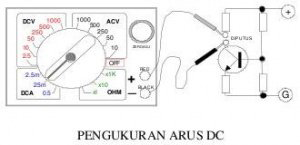DC Current Measurement

#### Measuring Resistance

The point of measuring resistance is to determine the condition of a component in a damaged or good state, and to determine how much the value of resistance is. Suppose a resistor has a color code: brown, black, red and gold tolerance means that the resistor has a resistance value of 1000 ohms with a tolerance of 5%, meaning that the resistor is still good if after measuring the value it is still between +/- 5% of 1000 ohms, or between 950 to 1050 ohms. How to measure it as follows: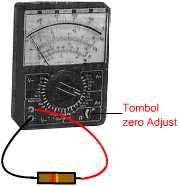Measuring Resistors
1. Set the selector switch to the ohms position
2. Select the measuring limit (range) whether: x1, x10, x100, or x1000 (adjust to the resistor value)
3. Firstly, short circuit the probe so that the meter needle moves to the right and can be adjusted to show the maximum scale by turning the Zero Adjust knob, meaning that the meter reading is in accordance with the scale and range used.
4. Start measuring the resistor by connecting the probe wires on the two legs of the resistor in parallel, regardless of the wire color
5. Read the scale board according to where the meter needle stopped, and multiply the reading by the measuring limit. For example, the needle shows on a scale of 10 and the measurement limit uses x 100, then the resistor value is 1000 ohms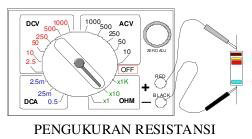Resistance Measurement

## Chapter 4 Closing

### 4.1 Conclusion

AVO meter is a combination of ammeter (ammeter), voltmeter, ohmmeter made into 1 tool. Tools that have varied functions, namely measuring current, voltage and resistance, are often termed a multitester. In fact, the author wants to explain in detail about the AVO meter. The real writer has the aim of explaining the definition, how to use it, how it works, so that the reader can make the AVO meter itself. By understanding how to make it the reader can repair it if it is damaged. However, it is unfortunate that limited time and knowledge prevent the writer from achieving this goal. Hopefully this assignment is useful for all who read and apologize if there are errors. In the end let me say thank you.